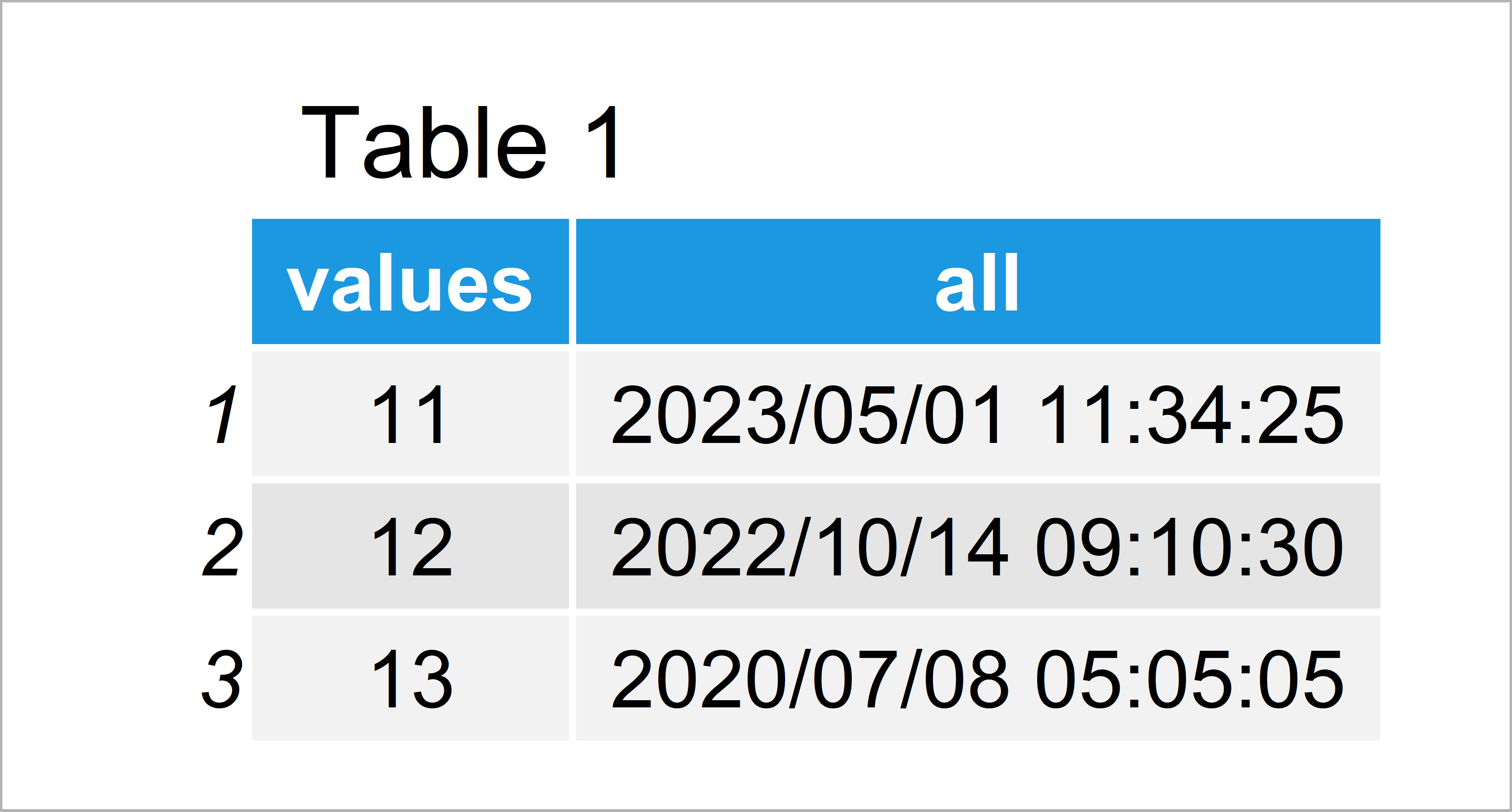# How to Split a Date-Time Column into Separate Variables in R (Example)

This tutorial illustrates how to divide a date-time object into two different variables in R programming.

The tutorial will consist of one example for the splitting of date-times. To be more precise, the post looks as follows:

So now the part you have been waiting for – the example.

## Example Data

To begin with, let’s construct some example data in R:

```data <- data.frame(values = 11:13, # Create example data frame all = c("2023/05/01 11:34:25", "2022/10/14 09:10:30", "2020/07/08 05:05:05")) data # Print example data frame```Table 1 visualizes that our example data is made of three data points and two columns. The variable all contains a dates and times.

## Example: Add Date & Time Variables to Data Frame Using as.Date, format & as.POSIXct Functions

This example explains how to split our date-time column into two separate columns.

In a first step, we can use the as.Date function to add a date variable to our data frame:

`data\$date <- as.Date(data\$all) # Add date column`

Next, we can apply the format and as.POSIXct functions to add a time variable to our data:

```data\$time <- format(as.POSIXct(data\$all), # Add time column format = "%H:%M:%S")```

Let’s have a look at our updated data frame:

`data # Print updated data frame`Table 2 reveals the output of the previous R syntax: Our input data frame plus a date and a time column.

## Video, Further Resources & Summary

Do you want to learn more about dates and times in R? Then you could watch the following video of my YouTube channel. In the video, I illustrate the contents of this page in RStudio.

In addition, you might have a look at the related articles on my website. Some tutorials can be found below.

In this R tutorial you have learned how to separate a date-time data object. Don’t hesitate to tell me about it in the comments section, in case you have further questions.

Subscribe to the Statistics Globe Newsletter